Donsker invariance principle

A principle [a1] stating that under some conditions the distribution of a functional of normalized sums,, of independent and identically distributed random variables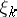converges to the distribution of this functional of the Wiener process.

Donsker's theorem is as follows [a2]. Suppose the random variables,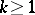, are independent and identically distributed with meanand finite, positive variance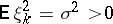(cf. also Random variable).

Then the random continuous functions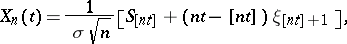(a1)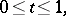converge weakly (cf. also Weak convergence of probability measures) to the Wiener process: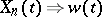; that is, for every bounded and continuous real-valued functionalon the space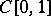of continuous functions on the interval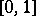, with the uniform topology, the weak convergence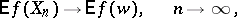(a2)

takes place; equivalently, for an arbitrary setin the Borel-algebrainwith, one has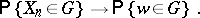(a3)

For the more general case of a triangular array with, in every line, independent random variables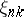,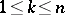, and under the conditions of the Lindeberg–Levy central limit theorem (cf. also Central limit theorem), the weak convergence in (a2) for sums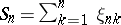,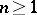, is called the Donsker–Prokhorov invariance principle [a3], [a4].

The notion of "invariance principle" is applied as follows. The sums,,, can be interpreted as positions of a random walk. The convergence (a2) means that all trajectories are trajectories of a Brownian motion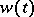,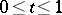, whenis large enough. This is the reason that the invariance principle is also called the functional central limit theorem.

An important application of the invariance principle is to prove limit theorems for various functions of the partial sums, for example,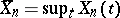,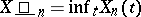,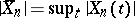, etc.

The independence of the limiting distribution from the distribution of the random terms enables one to compute the limit distribution in certain easy special cases. For example, the distributioncan be calculated from the partial sums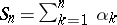,, of independent and identically Bernoulli-distributed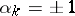with probabilities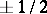by using the reflection principle [a2]. This argument follows a general pattern. Ifis a continuous functional on(or continuous except at points forming a set of Wiener measure), then one can find the distribution of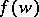by finding the explicit distribution of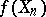, which is the distribution ofby the invariance principle.

The idea of computing the limiting distribution by using a special case and then passing to the general case was first realized by by A. Kolmogorov (1931) and subsequently for the various particular cases by P. Erdös and M. Kac (1946; [a6]). Kolmogorov and Yu.V. Prokhorov (1954) were the first to point out the weak convergence (a2). Much work has been done in connection with the estimation of the rate of convergence in the invariance principle (see, for example, [a5]).